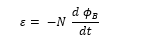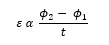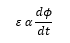Michael Faraday demonstrated the reverse effect of Oersted experiment. He explained the possibility of producing emf across the ends of a conductor when the magnetic flux linked with the conductor changes. This was termed as electromagnetic induction. The discovery of this phenomenon brought about a revolution in the field of power generation.

### Faraday’s Laws of electromagnetic induction

Faraday’s laws of induction imply that the emf induced in a coil of N turns is directly related to the rate of change of flux through it,Here ϕB is the flux linked with one turn of the coil. If the circuit is closed, a current I = ε/R is set up in it, where R is the resistance of the circuit.

Faraday proposed the following two laws:

Whenever the amount of magnetic flux linked with a closed circuit changes, an emf is induced in the circuit. The induced emf lasts so long as the change in magnetic flux continues.

The magnitude of emf induced in a closed circuit is directly proportional to the rate of change of magnetic flux linked with the circuit.

Let ϕ1 be the magnetic flux linked to the coil initially and ϕ2 be the magnetic flux linked with the coil after a time t. ThenAccording to Faraday’s second law, the magnitude of induced emf is,If dϕ is the change in magnitude flux in a time dt, then the above equation can be written as